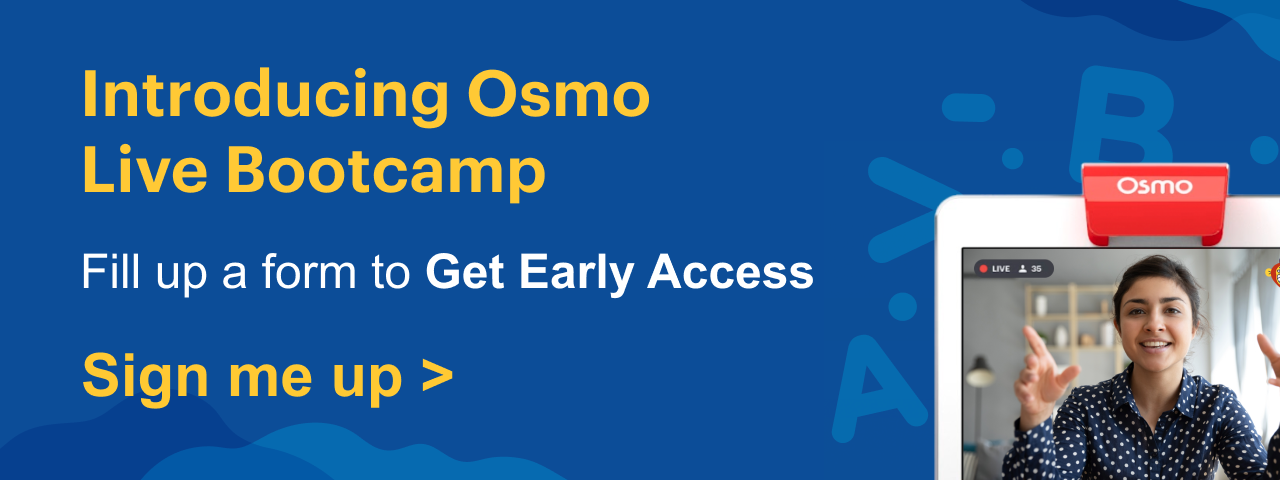# Triangle Worksheet

Introduce your child to triangles using a triangle worksheet. The world around us is filled with wonderful shapes, everything from plates, cups, mirrors to nacho chips has a distinct shape. Geometry is the study of some of these fascinating shapes and triangles are one of them. Teaching children about shapes from an early age sets a solid foundation for geometry learning later. Use shape games for kids to boost your child’s learning of shapes. Triangles are simple polygons with three sides and three angles and 3 corners. Help your child learn and understand all about this 3 sided figure with a triangle worksheet.

The study of triangles, called trigonometry, is an important branch of geometry. It has been around for more than 2000 years. The triangle might be a simple shape, but it is one of the most important shapes in geometry. They’re also the strongest of all the geometric shapes. Of all the 2-dimensional shapes you can make out of metal, a triangle is the most rigid and the strongest shape. One of the easiest ways to help kids learn this concept is by solving math worksheets for kids. Use the triangle worksheet to help your child learn about the 3 sided polygon easily.

## Free Printable Triangle Worksheet

A triangle is a closed, two-dimensional polygon that has three sides, three angles and three corners. Geometry lessons can sometimes be too complex and confusing for kids to understand. Clear the confusion and make learning about triangles fun and easy with these worksheets for kids on triangles.

### Triangle Worksheet for Preschool

It never hurts to help children learn geometry early. By the time kids start preschool, they start recognizing shapes and patterns around them. Learning about the basic shapes helps children identify and organize visual information. Shapes are also important for children to learn about alphabets, numbers, signs and symbols. Learning shapes also helps them understand concepts like size, space and position.

Math is an important life skill kids need to learn, and learning shapes lays the groundwork for that. Help your preschooler identify a triangle and draw it using this triangle worksheet for kids in preschool.

Preschool is a great time to introduce kids to some of the basic geometric shapes using preschool shape worksheets.

A right triangle or a right-angled triangle is a triangle with one right angle and two acute angles. The area of a triangle is the space enclosed within the three sides of the triangle. Here is the formula to measure the area of a right triangle:

Area of the triangle = ½ x base x height

The longest side of a triangle is called the hypotenuse. The other two sides are called legs or the base and the height of the triangle. The height of the triangle is on the opposite side of the hypotenuse and the base is the bottom. Practice calculating the area, missing sides and angles of a right triangle using these right triangle worksheets.

### Find the Area in this Triangle Worksheet

To upgrade your children’s knowledge on triangle, you can teach the how to find the area of a triangle using the worksheets. These worksheet help children solve problems effectively. Kids need to use the formula to find the area of a triangle on the worksheet given below.

## Benefits of Solving Triangle Worksheet for Kids

Wondering why your child should learn about triangles and solve triangle worksheets? Here are a few benefits of learning about triangles and solving worksheets for kids on triangles.

• Triangles are everywhere around us: These 3 sided polygons are special because they’re fundamental for our physical, mathematical and even the digital world. Triangles are an important feature in architecture and construction, creating CGI characters, calculating the height of mountains and cutting pizza and sandwiches.
• Useful for navigating ships, studying stars and planets: Trigonometry, the branch of math that studies triangles, is very helpful. It is necessary to know about triangles to study constellations, stars and planets. It is also very important for designing and navigating ships and airplanes and to determine altitude, position and land boundaries. Triangles are also an important feature in construction, architecture and even medical procedures like MRI And CAT scans.
• Practice Problems: Once your child learns about triangles, solving worksheets on triangles help reinforce the knowledge. The more problems they solve, the more confident they get in the subject.

Looking for more math activities, games or worksheets? Check Osmo for more activities, games and worksheets to aid in your kids learning. Check out this 3D shapes worksheet once your child has learnt about 2D shapes.

## Frequently Asked Questions on Triangle Worksheet

### What is a triangle?

A triangle is a closed, 3-sided polygon with 3 angles, 3 sides and 3 vertices.

### How many types of triangles are there and what are they?

There are 7 different types of triangles. The types of triangles are equilateral, right isosceles, obtuse isosceles, acute isosceles, right scalene, obtuse scalene, and acute scalene.

 Kids Learning Related Links Printable Horse Coloring Pages Weather Chart For Kids Multiplication Table Worksheet 11 To 20 Table Chart Fall Poems For Kids Flower Craft For Kids Printable Abc Tracing 21 To 30 Tables Chart